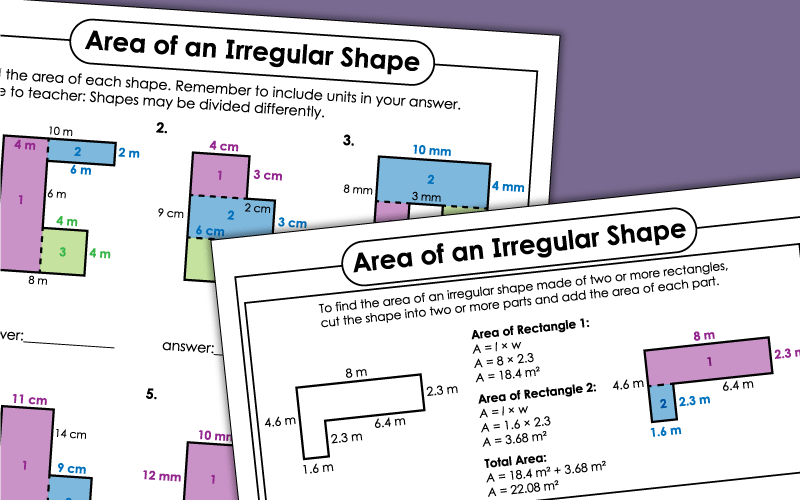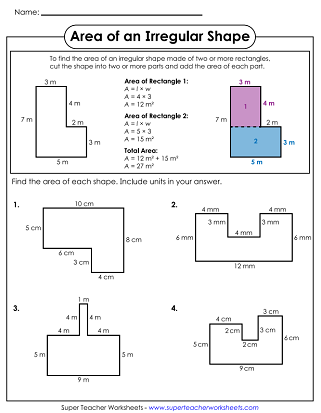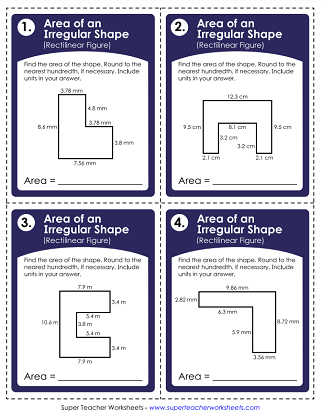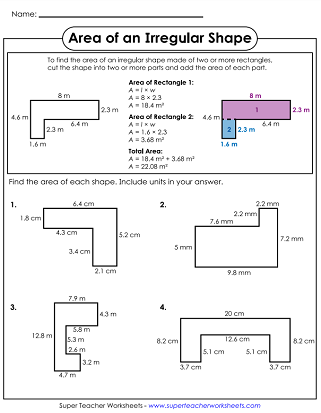# Areas of Irregular Shapes (Rectilinear Figures)

Download and print these worksheets to help students learn to find areas of rectilinear figures (irregular shapes made up of 2 or more rectangles).## Basic

Find the area of the irregular shapes (rectilinear figures) by finding the areas of the individual rectangles and adding them together.
First, multiply to find the areas of the individual rectangles. Then, add them together to find the area of the irregular shape (rectilinear figure).
Use these printable task cards to find the areas of irregular shapes (rectilinear figures). Find the area of each smaller shape by multiplying whole numbers and then add those together.

Multiply decimals to find the areas of each rectangle that makes up the irregular shape (rectilinear figure). Then add those together to find the total area.
Find the area of the irregular shape (rectilinear figure) by finding the areas of each rectangle within the shape and then adding those together.
Find the area of these irregular shapes (rectilinear figures) by multiplying decimals to the tenths and hundredths place. This is a class set of 30 task cards.
More Area Worksheets

Areas of Rectangles and Squares

Have your students practice finding the areas of squares and rectangles with these printables.

## Sample Worksheet ImagesMy Account
Site Information